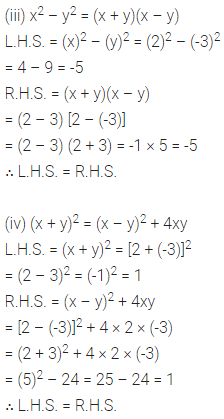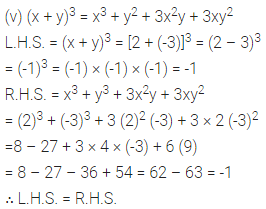# ML Aggarwal Class 6 Solutions for ICSE Maths Chapter 9 Algebra Ex 9.4

## ML Aggarwal Class 6 Solutions for ICSE Maths Chapter 9 Algebra Ex 9.4

Question 1.
Find the value of the following:
(i) 43
(ii) (-6)4
(iii) $$\left(\frac{2}{3}\right)^{4}$$
(iv) (-2)3 × 52
Solution:Question 2.
Find the value of:
(i) 3x + 2y when x = 3 and y – 2
(ii) 5x – 3y when x = 2 and y = -5
(iii) a + 2b – 5c when a = 2, b = -3 and c = 1
(iv) 2p + 3q + 4r + pqr when p = -1, q = 2 and r = 3
(v) 3ab + 4bc – 5ca when a = 4, 6 = 5 and c = -2.
Solution: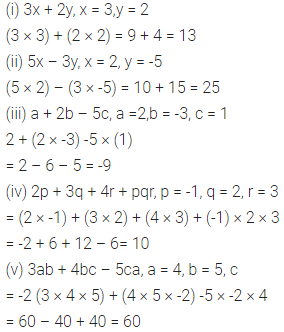Question 3.
Find the value of:
(i) 2x2 – 3x + 4 when × = 2
(ii) 4x3 – 5x2 – 6x + 7 when x = 3
(iii) 3x3 + 9x2 – x + 8 when x = -2
(iv) 2x4 – 5x3 + 7x – 3 when x = -3
Solution:Question 4.
If x = 5, find the value of:
(i) 6 – 7x2
(ii) 3x2 + 8x – 10
(iii) 2x3 – 4x2 – 6x + 25
Solution: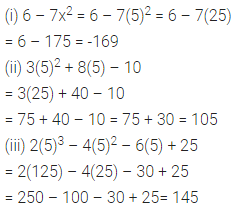Question 5.
If x = 2, y = 3 and z = -1, find the values of:
(i) x + y
(ii) $$\frac{x y}{z}$$
(iii) $$\frac{2 x+3 y-4 z}{3 x-z}$$
Solution: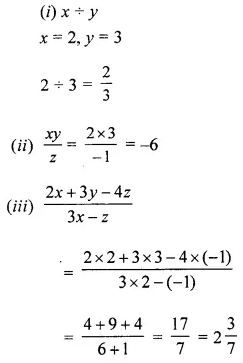Question 6.
If a = 2, b = 3 and c = -2, find the value of a2 + b2 + c2 – 2ab – 2bc – 2ca + 3abc.
Solution: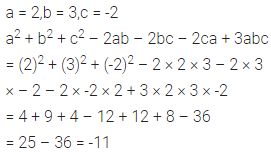Question 7.
If p = 4, q = -3 and r = 2, find the value of: p3 + q3 – r3 – 3pqr.
Solution: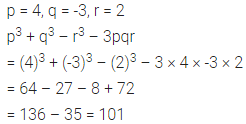Question 8.
If m = 1, n = 2 and p = -3, find the value of 2mn4 – 15m2n + p.
Solution: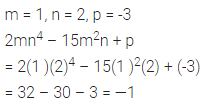Question 9.
State true or false:
(i) The value of 3x – 2 is 1 when x = 0.
(ii) The value of 2x2 – x – 3 is 0 when x = -1.
(iii) p2 + q2 – r2 when p = 5, q = 12 and r = 13.
(iv) 16 – 3x = 5x when x = 2.
Solution:Question 10.
For x = 2 and y = -3, verify the following:
(i) (x + y)2 = x2 + 2xy + y2
(ii) (x – y)2 = x2 – 2xy + y2
(iii) x2 – y2 = (x + y) (x – y)
(iv) (x + y)2 = (x – y)2 + 4xy
(v) (x + y)3 = x3 + y3 + 3x2y + 3xy2
Solution: